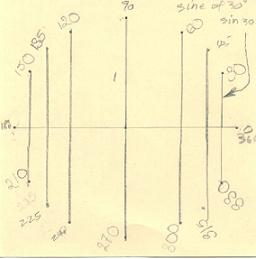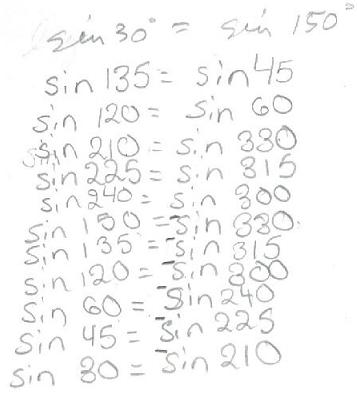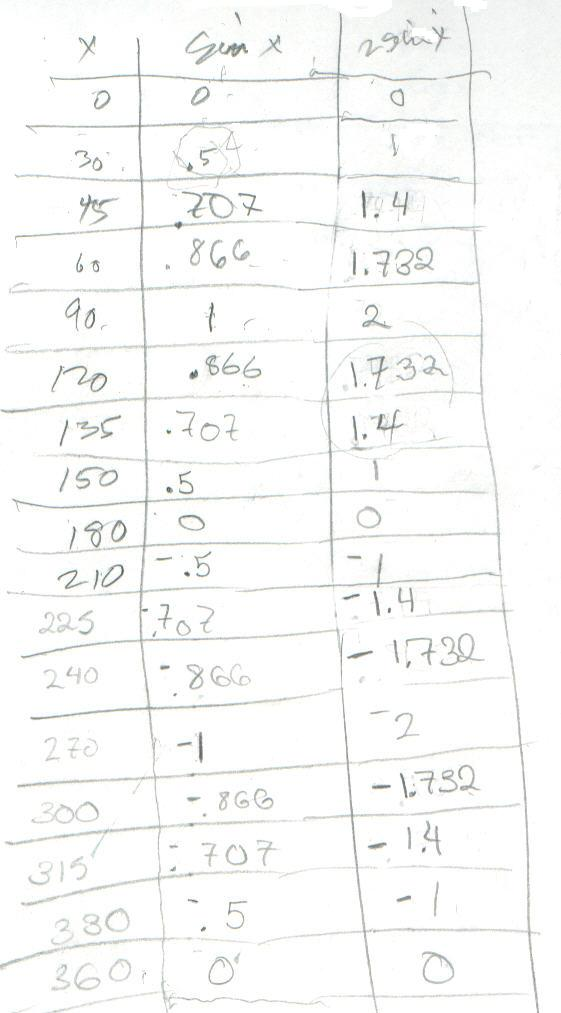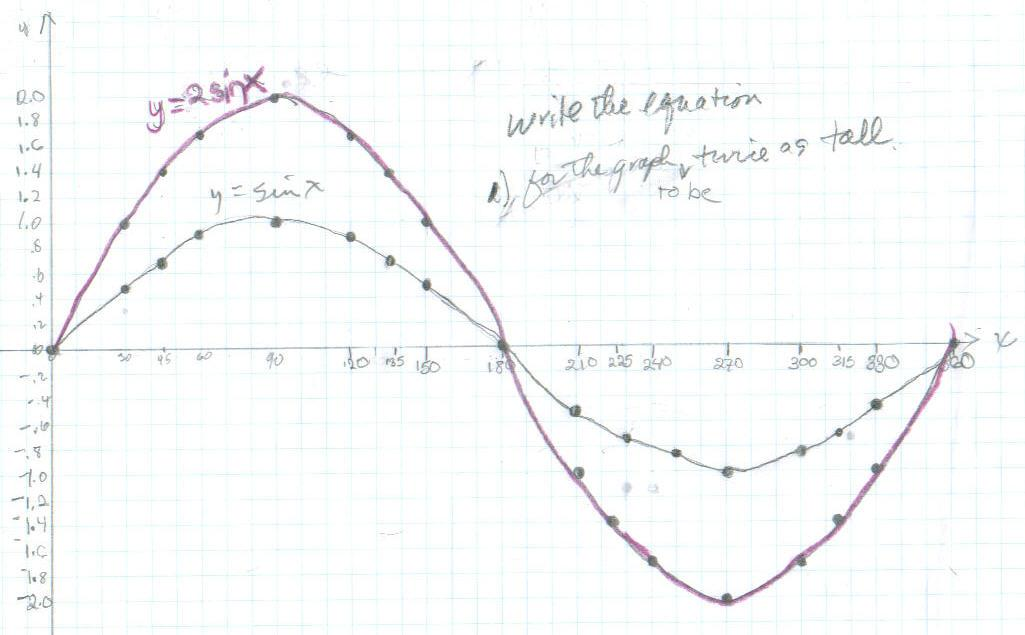Sheri, a 5th grader, begins trig

This is a 12 point circle.  All of the points  are 30 degrees

apart. Then Mr. Cohen added the points at 45' around.  Each of them are equal to one other point on the circle.All of these  are equal to each other:[Sheri measured the lengths of the segments for the sines of 30', 45', and 60', then found the ratios of these to the measured distance for 90' and came out very close to the calculator values in the table below for sin x.]I graphed y=sin x, then found the equation for the graph to be twice as tall ( y= 2 sin x); I multiplied each sine value by 2".Don went over things like 2x.7 = 1.4 which Sheri wasn't sure about. But it was her idea to double sin x to get the equation of the graph to be twice as tall. Fine job Sheri!

Sheri writes 64 using exponents
Sheri solves the quadratic equation x2 - x - 1 = 0
Sheri finds the base for Don's age of   114? = 7110
Sheri uses the quadratic formula to find base for Don's age of   114? = 7110
Sheri finds the measure of an inscribed angle
Sheri uses binary numerals to make the Magic Number Game cards
Sheri changes the shape of a dog using matrices
Sheri reduces the size of a shell using the pantograph
Sheri moves a parabola and finds the equation
Sheri finds the ratio of The Volume of a Pyramid / The Volume of a Cube (3 ways)
Sheri works with the sand pendulum
Sheri figures out a rule for The Tower Puzzle

To other discoveries

To order Don's materials
Mathman home